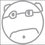# What is 0/0?

Anything 0/x= 0
Anything x/0=unidentified
Anything x/x= 1
0/0 * 0/0=1
0 * unidentified=1
What is happening here!?!?!?!
Anything * 0=0
0 * 1=0
Divide by 0
1=0/0?Note by Juice Guy
1 year, 1 month ago

This discussion board is a place to discuss our Daily Challenges and the math and science related to those challenges. Explanations are more than just a solution — they should explain the steps and thinking strategies that you used to obtain the solution. Comments should further the discussion of math and science.

When posting on Brilliant:

• Use the emojis to react to an explanation, whether you're congratulating a job well done , or just really confused .
• Ask specific questions about the challenge or the steps in somebody's explanation. Well-posed questions can add a lot to the discussion, but posting "I don't understand!" doesn't help anyone.
• Try to contribute something new to the discussion, whether it is an extension, generalization or other idea related to the challenge.

MarkdownAppears as
*italics* or _italics_ italics
**bold** or __bold__ bold
- bulleted- list
• bulleted
• list
1. numbered2. list
1. numbered
2. list
Note: you must add a full line of space before and after lists for them to show up correctly
paragraph 1paragraph 2

paragraph 1

paragraph 2

[example link](https://brilliant.org)example link
> This is a quote
This is a quote
    # I indented these lines
# 4 spaces, and now they show
# up as a code block.

print "hello world"
# I indented these lines
# 4 spaces, and now they show
# up as a code block.

print "hello world"
MathAppears as
Remember to wrap math in $$ ... $$ or $ ... $ to ensure proper formatting.
2 \times 3 $2 \times 3$
2^{34} $2^{34}$
a_{i-1} $a_{i-1}$
\frac{2}{3} $\frac{2}{3}$
\sqrt{2} $\sqrt{2}$
\sum_{i=1}^3 $\sum_{i=1}^3$
\sin \theta $\sin \theta$
\boxed{123} $\boxed{123}$

Sort by:

$\frac{0}{0}$ is what's known as indeterminate form. This may seem confusing at the start, but it makes more sense once you try to use slopes of lines.

Consider a line that contains two points, namely $(x_{1}, y_{1})$ and $(x_{2}, y_{2})$. The slope of the function is $\frac{y_{2}-y_{1}}{x_{2}-x_{1}}$. Now, it doesn't matter which two points we choose: as long as they are on the same line, we should get the same slope, right? Well, that gives us an idea: what if those two points are the same? Then, our slope would be $\frac{y_{1}-y_{1}}{x_{1}-x_{1}}=\frac{0}{0}$. We expected to get the same slope, but we got $\frac{0}{0}$. This is because there are infinite number of lines that pass through a specific point. We don't know which line we are talking about, or in other words, we can't determine which line we are talking about, hence the name indeterminate form.

This example shows that $\frac{0}{0}$ is not undefined. If it was undefined, we would be sure that our line is vertical. At the same time, it shows that $\frac{0}{0}$ is not zero. Otherwise, we would be sure that our line is horizontal. $\frac{0}{0}$ just means that we don't know enough or have enough information to know specifically what it is; it could be anything. Thus, we call it indeterminate form.

There are other examples of indeterminate forms: $0^0$, $\frac{\infty}{\infty}$, $0 \times \infty$, and more. It's a very interesting subject. You'll learn more about indeterminate forms when you learn about limits in precalculus.

- 1 year, 1 month ago

Cool, but I'm still confused.

- 1 year, 1 month ago

@Juice Guy : Here is a good resource on indeterminate forms: https://brilliant.org/wiki/indeterminate-forms/. If you are still confused, don't worry. You don't need to completely understand it to to see why it's important. Just make sure that whenever you do any arithmetic and algebra, don't divide by zero. And if you are using limits (like in calculus or precalculus), you need to simplify to get out of indeterminate form.

- 1 year, 1 month ago

Thanks!

- 1 year, 1 month ago

You're welcome!

- 1 year, 1 month ago

Suppose we naively assume that $\frac{0}{0}$ has some defined value; call it $a$. We then run into a problem, since zero multiplied by anything is zero.

$\frac{0}{0} = a = \frac{b \, 0}{c \, 0 } = \frac{b}{c} a$

For $b \neq c$, the statement above is inconsistent. So you have to choose some property of zero to throw away. We cant throw away the property that zero multiplied by a number is zero (it is too useful), so we have to throw away the "zero over zero" property instead.

- 1 year, 1 month ago

That is so cool!

- 1 year, 1 month ago